# Difference AP

Calculate the difference of arithmetic progression if the sum of its first 19 members Sn = 8075 and the first member is a1 = 20

d =  45

### Step-by-step explanation: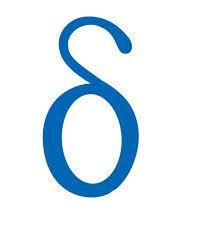Did you find an error or inaccuracy? Feel free to write us. Thank you!## Related math problems and questions:

• Fifth memberDetermine the fifth member of the arithmetic progression, if the sum of the second and fifth members equal to 73, and difference d = 7.
• Geometric progressionIn geometric progression, a1 = 7, q = 5. Find the condition for n to sum first n members is sn≤217.
• Arithmetic progression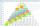In some AP applies: 5a2 + 7a5 = 90 s3 = 12 Find the first member a =? and difference d = ?
• AP members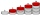What is the value of x2, x3, x4, x5…of the terms (of arithmetic progression) when x1 = 8 and x6 = 20?
• The sumThe sum of the first 10 members of the arithmetic sequence is 120. What will be the sum if the difference is reduced by 2?
• Difference AP 4Calculate the difference of the AP if a1 = 0.5, a2 + a3 = -1.1
• Third memberDetermine the third member of the AP if a4=93, d=7.5.
• Common difference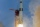The 4th term of an arithmetic progression is 6. If the sum of the 8th and 9th terms is -72, find the common difference.
• AP 6Calculate the first five items of an arithmetic sequence if it is given: a2 – a3 + a5 = 20 a1 + a6 = 38
• Sequences AP + GPThe three numbers that make up the arithmetic sequence have the sum of 30. If we subtract from the first 5, from the second 4 and keep the third, we get the geometric sequence. Find AP and GP members.
• Geometric sequenceIn the geometric sequence is a4 = 20 a9 = -160. Calculate the first member a1 and quotient q.
• Sum of membersWhat is the sum of the first two members of the aritmetic progression if d = -4.3 and a3 = 7.5?
• GP - three membersThe second and third of a geometric progression are 24 and 12(c+1), respectively, given that the sum of the first three terms of progression is 76. determine the value of c.
• Determine AP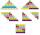Determine the difference of the arithmetic progression if a3 = 7, and a4 + a5 = 71
• Nice prismCalculate the cuboid's surface if the sum of its edges is a + b + c = 19 cm and the body diagonal size u = 13 cm.
• Rectangle fieldThe field has a shape of a rectangle having a length of 119 m and a width of 19 m. , How many meters have to shorten its length and increase its width to maintain its area and circumference increased by 24 m?
• Negative differenceFind four arithmetic progression members between 7 and -6.